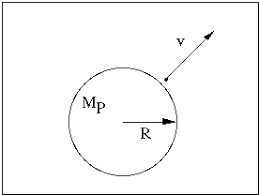## How to Calculate and Solve for Escape Velocity | The Calculator EncyclopediaThe image above represents the escape velocity.

To compute the escape velocity of a field, two essential parameters are needed and the parameters are acceleration due to gravity (g) and radius (r).

The formula for calculating the escape velocity:

V = √(2gR)

Where;
V = Escape velocity
g = Acceleration due to gravity

Let’s solve an example;
Find the escape velocity of a field when the acceleration due to gravity is 12 and the radius is 24 cm.

This implies that;
g = Acceleration due to gravity = 12
r = Radius = 24 cm

V = √(2gR)
V = √(2 x 12 x 24)
V = √(576)
V = 24

Therefore, the escape velocity is 24 m/s.